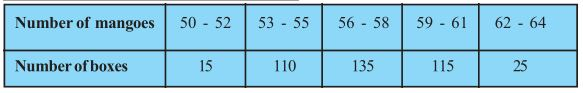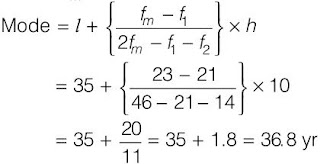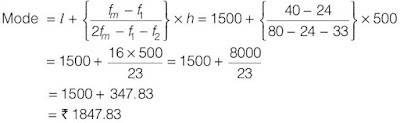# NCERT Solutions for Class 10th: Ch 14 Statistics Maths

#### NCERT Solutions for Class 10th: Ch 14 Statistics Maths

Page No: 270

Exercise 14.1

1. A survey was conducted by a group of students as a part of their environment awareness programme, in which they collected the following data regarding the number of plants in 20 houses in a locality. Find the mean number of plants per house.
 Number of Plants 0-2 2-4 4-6 6-8 8-10 10-12 12-14 Number of Houses 1 2 1 5 6 2 3
Which method did you use for finding the mean, and why?

 No. of plants   (Class interval) No. of houses (fi) Mid-point (xi) fixi 0-2 1 1 1 2-4 2 3 6 4-6 1 5 5 6-8 5 7 35 8-10 6 9 54 10-12 2 11 22 12-14 3 13 39 Sum fi = 20 Sum fixi = 162

Mean = x̄ = ∑fixi /f= 162/20 = 8.1

We would use direct method because the numerical value of fi and xi are small.

2. Consider the following distribution of daily wages of 50 workers of a factory.
 Daily wages (in Rs.) 100-120 120-140 140-160 160-180 180-200 Number of workers 12 14 8 6 10
Find the mean daily wages of the workers of the factory by using an appropriate method.

Here, the value of mid-point (xi) is very large, so assumed mean A = 150 and class interval is h = 20.
So, u= (xi - A)/h = u= (xi - 150)/20

 Daily wages   (Class interval) Number of workersfrequency (fi) Mid-point (xi) ui = (xi - 150)/20 fiui 100-120 12 110 -2 -24 120-140 14 130 -1 -14 140-160 8 150 0 0 160-180 6 170 1 6 180-200 10 190 2 20 Total Sum fi = 50 Sum fiui = -12
Mean = x̄ = A + h∑fiui /f=150 + (20 × -12/50) = 150 - 4.8 = 145.20
Thus, mean daily wage = Rs. 145.20

3. The following distribution shows the daily pocket allowance of children of a locality. The mean pocket allowance is Rs 18. Find the missing frequency f.Here, the value of mid-point (xi)  mean  = 18

 Class interval Number of children (fi) Mid-point (xi) fixi 11-13 7 12 84 13-15 6 14 84 15-17 9 16 144 17-19 13 18 = A 234 19-21 f 20 20f 21-23 5 22 110 23-25 4 24 96 Total fi = 44+f Sum fixi = 752+20f

Mean = x̄ = ∑fixi /f= (752+20f)/(44+f)
⇒ 18 = (752+20f)/(44+f)
⇒ 18(44+f) = (752+20f)
⇒ 792+18f = 752+20f
⇒ 792+18f = 752+20f
⇒ 792 - 752 = 20f - 18f
⇒ 40 = 2f
⇒ f = 20

Page No: 271

4. Thirty women were examined in a hospital by a doctor and the number of heart beats per minute were recorded and summarised as follows. Find the mean heart beats per minute for these women, choosing a suitable method.x= (Upper limit + Lower limit)/2
Class size (h) = 3
Assumed mean (A) = 75.5

 Class Interval Number of women (fi) Mid-point (xi) ui = (xi - 75.5)/h fiui 65-68 2 66.5 -3 -6 68-71 4 69.5 -2 -8 71-74 3 72.5 -1 -3 74-77 8 75.5 0 0 77-80 7 78.5 1 7 80-83 4 81.5 3 8 83-86 2 84.5 3 6 Sum fi= 30 Sum fiui = 4

Mean = x̄ = A + h∑fiui /f= 75.5 + 3×(4/30) = 75.5 + 4/10 = 75.5 + 0.4 = 75.9
The mean heart beats per minute for these women is 75.9

5. In a retail market, fruit vendors were selling mangoes kept in packing boxes. These boxes contained varying number of mangoes. The following was the distribution of mangoes according to the number of boxes.Find the mean number of mangoes kept in a packing box. Which method of finding the mean did you choose?

Since, the given data is not continuous so we add 0.5 to the upper limit and subtract 0.45 from the lower limit.
Here, assumed mean (A) = 57
Class size (h) = 3

 Class Interval Number of boxes (fi) Mid-point (xi) di = xi - A fidi 49.5-52.5 15 51 -6 90 52.5-55.5 110 54 -3 -330 55.5-58.5 135 57 = A 0 0 58.5-61.5 115 60 3 345 61.5-64.5 25 63 6 150 Sum fi = 400 Sum fidi = 75

Mean = x̄ = A + ∑fidi /f= 57 + (75/400) = 57 + 0.1875 = 57.19

6. The table below shows the daily expenditure on food of 25 households in a locality.Find the mean daily expenditure on food by a suitable method.

Here, assumed mean (A) = 225

 Class Interval Number of households (fi) Mid-point (xi) di = xi - A fidi 100-150 4 125 -100 -400 150-200 5 175 -50 -250 200-250 12 225 0 0 250-300 2 275 50 100 300-350 2 325 100 200 Sum fi = 25 Sum fidi = -350

Mean = x̄ = A + ∑fidi /f= 225 + (-350/25) = 225 - 14 = 211
The mean daily expenditure on food is 211

7. To find out the concentration of SO2 in the air (in parts per million, i.e., ppm), the data was collected for 30 localities in a certain city and is presented below:Find the mean concentration of SO2 in the air.

 Concentration of SO2 (in ppm) Frequency (fi) Mid-point (xi) fixi 0.00-0.04 4 0.02 0.08 0.04-0.08 9 0.06 0.54 0.08-0.12 9 0.10 0.90 0.12-0.16 2 0.14 0.28 0.16-0.20 4 0.18 0.72 0.20-0.24 2 0.20 0.40 Total Sum fi = 30 Sum (fixi) = 2.96

Mean = x̄ = ∑fixi /fi
= 2.96/30 = 0.099 ppm

Page No. 272

8. A class teacher has the following absentee record of 40 students of a class for the whole
term. Find the mean number of days a student was absent.

 Number of students Number of days 0-6 6-10 10-14 14-20 20-28 28-38 38-40 11 10 7 4 4 3 1

 Class interval Frequency (fi) Mid-point (xi) fixi 0-6 11 3 33 6-10 10 8 80 10-14 7 12 84 14-20 4 17 68 20-28 4 24 96 28-38 3 33 99 38-40 1 39 39 Sum fi = 40 Sum fixi = 499

Mean = x̄ = ∑fixi /fi
= 499/40 = 12.48 days

9. The following table gives the literacy rate (in percentage) of 35 cities. Find the mean
literacy rate.

 Literacy rate (in %) 45-55 55-65 65-75 75-85 85-98 Number of cities 3 10 11 8 3

 Class Interval Frequency (fi) (xi) di = xi - a ui = di/h fiui 45-55 3 50 -20 -2 -6 55-65 10 60 -10 -1 -10 65-75 11 70 0 0 0 75-85 8 80 10 1 8 85-95 3 90 20 2 6 Sum fi  = 35 Sum fiui  = -2

Mean = x̄ = a + (∑fiui /fi) х h
= 70 + (-2/35) х 10 = 69.42

Page No. 275

Exercise 14.2

1. The following table shows the ages of the patients admitted in a hospital during a year:

 Age (in years) 5-15 15-25 25-35 35-45 45-55 55-65 Number of patients 6 11 21 23 14 5

Find the mode and the mean of the data given above. Compare and interpret the two
measures of central tendency.

Modal class = 35 – 45, l = 35, class width (h) = 10, fm = 23, f1 = 21 and f2 = 14Calculation of Mean:

 Class Interval Frequency (fi) Mid-point (xi) fixi 5-15 6 10 60 15-25 11 20 220 25-35 21 30 630 35-45 23 40 920 45-55 14 50 700 55-65 5 60 300 Sum fi = 80 Sum fixi = 2830

Mean = x̄ = ∑fixi /fi
= 2830/80 = 35.37 yr

2. The following data gives the information on the observed lifetimes (in hours) of 225
electrical components :

 Lifetime (in hours) 0-20 20-40 40-60 60-80 80-100 100-120 Frequency 10 35 52 61 38 29

Determine the modal lifetimes of the components.

Modal class of the given data is 60–80.
Modal class = 60-80, l = 60, fm = 61, f1 = 52, f2 = 38 and h = 203. The following data gives the distribution of total monthly household expenditure of 200
families of a village. Find the modal monthly expenditure of the families. Also, find the
mean monthly expenditure :

 Expenditure Number of families 1000-1500 24 1500-2000 40 2000-2500 33 2500-3000 28 3000-3500 30 3500-4000 22 4000-4500 16 4500-5000 7